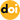Modeling and Feedback Control of LLC Resonant Converters at High Switching Frequency

Vol. 16, No. 3, pp. 849-860, May  201610.6113/JPE.2016.16.3.849

Abstract

The high-switching-frequency operation of power converters can achieve high power density through size reduction of passive components, such as capacitors, inductors, and transformers. However, a small-output capacitor that has small capacitance and low effective series resistance changes the small-signal model of the converter power stage. Such a capacitor can make the converter unstable by increasing the crossover frequency in the transfer function of the small-signal model. In this paper, the design and implementation of a high-frequency LLC resonant converter are presented to verify the power density enhancement achieved by decreasing the size of passive components. The effect of small output capacitance is analyzed for stability by using a proper small-signal model of the LLC resonant converter. Finally, proper design methods of a feedback compensator are proposed to obtain a sufficient phase margin in the Bode plot of the loop gain of the converter for stable operation at 500 kHz switching frequency. A theoretical approach using MATLAB, a simulation approach using PSIM, and experimental results are presented to show the validity of the proposed analysis and design methods with 100 and 500 kHz prototype converters.

Statistics
Show / Hide Statistics

Cumulative Counts from September 30th, 2019
Multiple requests among the same browser session are counted as one view. If you mouse over a chart, the values of data points will be shown.